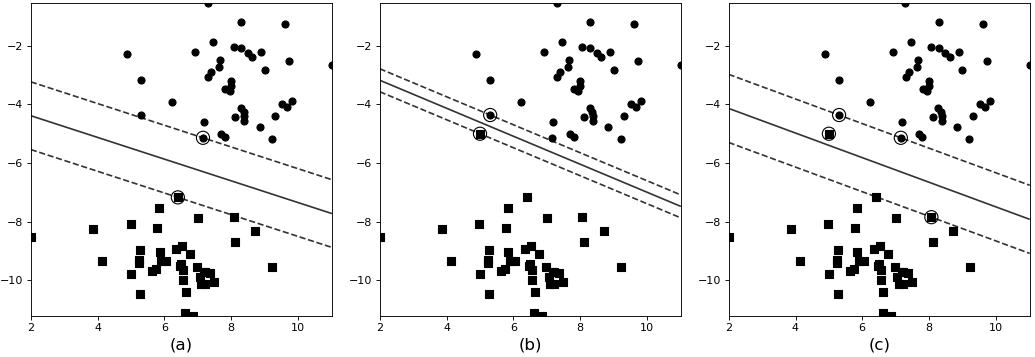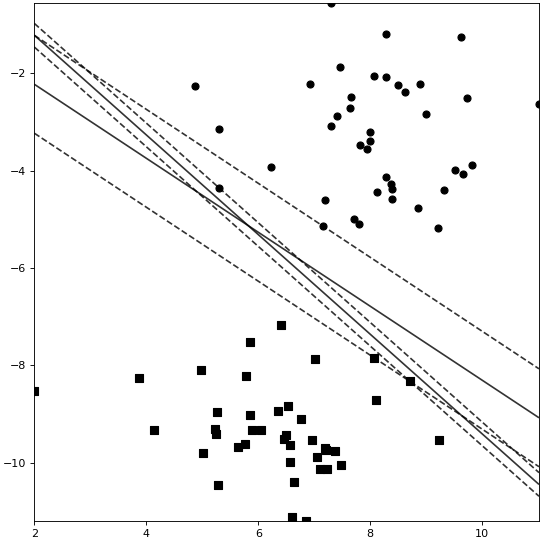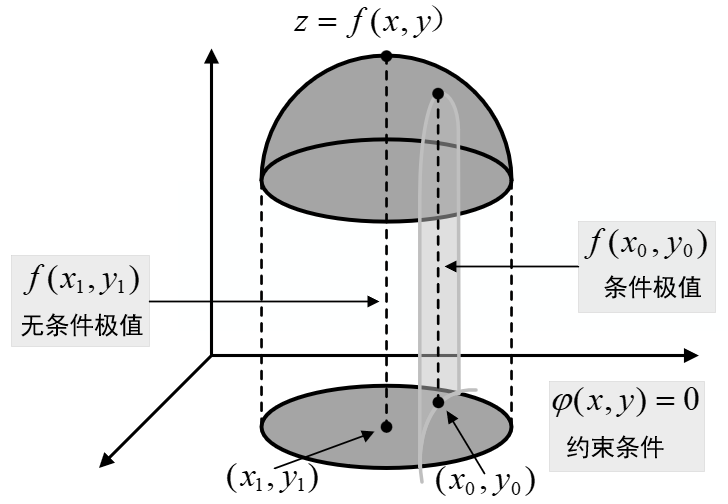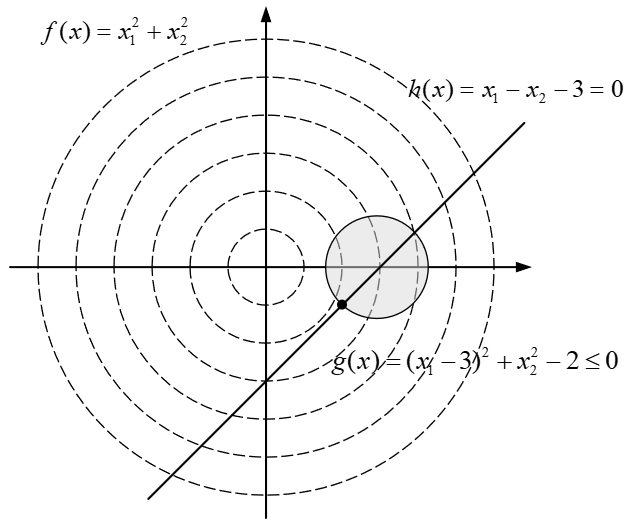### 1 SVM中的软间隔

#### 1.1 软间隔定义#### 1.2 最大化软间隔

${y}^{\left(i\right)}\left({w}^{T}{x}^{\left(i\right)}+b\right)\ge 1-{\xi }_{i}\phantom{\rule{0.278em}{0ex}}\phantom{\rule{0.278em}{0ex}}\phantom{\rule{0.278em}{0ex}}\phantom{\rule{0.278em}{0ex}}\phantom{\rule{0.278em}{0ex}}\phantom{\rule{0.278em}{0ex}}\phantom{\rule{0.278em}{0ex}}\phantom{\rule{0.278em}{0ex}}\phantom{\rule{0.278em}{0ex}}\left(1\right)$

#### 1.3 SVM软间隔示例代码

xxxxxxxxxx61def init(self,2    C=1.0, 3    kernel='rbf', 4    degree=3, 5    gamma='scale',6    coef0=0.0):• 模型选择

xxxxxxxxxx111def model_selection(x_train, y_train):2    model = SVC()3    paras = {'C': np.arange(1, 10, 5),4             'kernel': ['rbf', 'linear', 'poly'],5             'degree': np.arange(1, 10, 2),6             'gamma': ['scale', 'auto'],7             'coef0': np.arange(-10, 10, 5)}8    gs = GridSearchCV(model, paras, cv=3, verbose=2, n_jobs=3)9    gs.fit(x_train, y_train)10    print('best score:', gs.best_score_,'best 11                    parameters:', gs.best_params_)

xxxxxxxxxx71Fitting 3 folds for each of 240 candidates, totalling 720 fits2[CV] END ..C=1, coef0=-10,degree=1,gamma=scale,kernel=rbf;total time=  0.0s3[CV] END ..C=1, coef0=-10,degree=1,gamma=scale,kernel=rbf;total time=  0.0s4…5[CV] END ...C=6, coef0=5, degree=9,gamma=auto,kernel=rbf; total time= 0.5s6best score: 0.9867best parameters: {'C': 6, 'coef0': -10, 'degree': 1, 'gamma': 'scale', 'kernel': 'rbf'}

• 训练与预测

xxxxxxxxxx61def train(x_train, x_test, y_train, y_test):2    model = SVC(C=6, kernel='rbf',gamma='scale')3    model.fit(x_train, y_train)4    score = model.score(x_test, y_test)5    y_pred = model.predict(x_test)6    print("测试集上的准确率: ", score)# 0.985

### 2 拉格朗日乘数法

$F\left(x,y,\lambda \right)=xy+\lambda \left(x+y-1\right)\phantom{\rule{0.278em}{0ex}}\phantom{\rule{0.278em}{0ex}}\phantom{\rule{0.278em}{0ex}}\phantom{\rule{0.278em}{0ex}}\phantom{\rule{0.278em}{0ex}}\phantom{\rule{0.278em}{0ex}}\phantom{\rule{0.278em}{0ex}}\phantom{\rule{0.278em}{0ex}}\left(3\right)$

$\begin{array}{rl}& {F}_{x}=y+\lambda =0\\ & {F}_{y}=x+\lambda =0\\ & {F}_{\lambda }=x+y-1=0\end{array}\phantom{\rule{0.278em}{0ex}}\phantom{\rule{0.278em}{0ex}}\phantom{\rule{0.278em}{0ex}}\phantom{\rule{0.278em}{0ex}}\phantom{\rule{0.278em}{0ex}}\phantom{\rule{0.278em}{0ex}}\phantom{\rule{0.278em}{0ex}}\phantom{\rule{0.278em}{0ex}}\phantom{\rule{0.278em}{0ex}}\left(4\right)$

$x=\frac{1}{2};y=\frac{1}{2};\lambda =-\frac{1}{2}\phantom{\rule{0.278em}{0ex}}\phantom{\rule{0.278em}{0ex}}\phantom{\rule{0.278em}{0ex}}\phantom{\rule{0.278em}{0ex}}\phantom{\rule{0.278em}{0ex}}\phantom{\rule{0.278em}{0ex}}\phantom{\rule{0.278em}{0ex}}\phantom{\rule{0.278em}{0ex}}\phantom{\rule{0.278em}{0ex}}\left(5\right)$

#### 2.1 条件极值#### 2.2 求解条件极值

1) 作拉格朗日函数

$F\left(x,y,z,...,\lambda ,\mu ,...\right)=f\left(x,y,z,...\right)+\lambda \phi \left(x,y,...\right)+\mu \varphi \left(x,y,...\right)+...\phantom{\rule{0.278em}{0ex}}\phantom{\rule{0.278em}{0ex}}\phantom{\rule{0.278em}{0ex}}\phantom{\rule{0.278em}{0ex}}\phantom{\rule{0.278em}{0ex}}\phantom{\rule{0.278em}{0ex}}\left(6\right)$

2) 求多元函数$F\left(x,y,z,...,\lambda ,\mu ,...\right)$$F(x,y,z,...,\lambda,\mu,...)$的驻点

$\begin{array}{c}\left\{\begin{array}{l}{F}_{x}=0\\ {F}_{y}=0\\ \cdots \\ {F}_{\lambda }=0\\ \cdots \end{array}\phantom{\rule{0.278em}{0ex}}\phantom{\rule{0.278em}{0ex}}\phantom{\rule{0.278em}{0ex}}\phantom{\rule{0.278em}{0ex}}\phantom{\rule{0.278em}{0ex}}\phantom{\rule{0.278em}{0ex}}\phantom{\rule{0.278em}{0ex}}\phantom{\rule{0.278em}{0ex}}\left(7\right)\end{array}$

### 3 对偶性与KKT条件

#### 3.1 广义拉格朗日乘数法

$\mathcal{L}\left(w,\beta \right)=f\left(w\right)+\sum _{i=1}^{l}{\beta }_{i}{h}_{i}\left(w\right)\phantom{\rule{0.278em}{0ex}}\phantom{\rule{0.278em}{0ex}}\phantom{\rule{0.278em}{0ex}}\phantom{\rule{0.278em}{0ex}}\phantom{\rule{0.278em}{0ex}}\phantom{\rule{0.278em}{0ex}}\phantom{\rule{0.278em}{0ex}}\left(9\right)$

$\mathcal{L}\left(w,\alpha ,\beta \right)=f\left(w\right)+\sum _{i=1}^{k}{\alpha }_{i}{g}_{i}\left(w\right)+\sum _{i=1}^{l}{\beta }_{i}{h}_{i}\left(w\right)\phantom{\rule{0.278em}{0ex}}\phantom{\rule{0.278em}{0ex}}\phantom{\rule{0.278em}{0ex}}\phantom{\rule{0.278em}{0ex}}\phantom{\rule{0.278em}{0ex}}\phantom{\rule{0.278em}{0ex}}\phantom{\rule{0.278em}{0ex}}\left(11\right)$

#### 3.2 原始优化问题

${\theta }_{\mathcal{P}}\left(w\right)=\underset{\alpha ,\beta :{\alpha }_{i}\ge 0}{max}\phantom{\rule{0.167em}{0ex}}\mathcal{L}\left(w,\alpha ,\beta \right)\phantom{\rule{0.278em}{0ex}}\phantom{\rule{0.278em}{0ex}}\phantom{\rule{0.278em}{0ex}}\phantom{\rule{0.278em}{0ex}}\phantom{\rule{0.278em}{0ex}}\phantom{\rule{0.278em}{0ex}}\phantom{\rule{0.278em}{0ex}}\phantom{\rule{0.278em}{0ex}}\left(12\right)$

$\left(12\right)$$(12)$表示的含义是求得最大化$\mathcal{L}\left(w,\alpha ,\beta \right)$$\mathcal{L}(w,\alpha ,\beta )$$\alpha ,\beta$$\alpha,\beta$的取值，即$\alpha ,\beta$$\alpha,\beta$作为自变量与$w$$w$无关，最终求得的结果${\theta }_{\mathcal{P}}$$\theta_{\mathcal{P}}$是关于$w$$w$的函数。 因此，如果原约束条件${g}_{i}\left(w\right)\le 0$$g_i(w)\leq0$${h}_{i}\left(w\right)=0$$h_i(w)=0$均成立，那么式$\left(12\right)$$(12)$即等价为

$\underset{\alpha ,\beta :{\alpha }_{i}\ge 0}{max}\phantom{\rule{0.167em}{0ex}}\left[\sum _{i=1}^{k}{\alpha }_{i}{g}_{i}\left(w\right)+\sum _{i=1}^{l}{\beta }_{i}{h}_{i}\left(w\right)\right]\phantom{\rule{0.278em}{0ex}}\phantom{\rule{0.278em}{0ex}}\phantom{\rule{0.278em}{0ex}}\phantom{\rule{0.278em}{0ex}}\phantom{\rule{0.278em}{0ex}}\phantom{\rule{0.278em}{0ex}}\phantom{\rule{0.278em}{0ex}}\phantom{\rule{0.278em}{0ex}}\left(13\right)$

${p}^{\ast }=\underset{w}{min}\phantom{\rule{0.167em}{0ex}}{\theta }_{\mathcal{P}}\left(w\right)=\underset{w}{min}\phantom{\rule{0.167em}{0ex}}\underset{\alpha ,\beta :{\alpha }_{i}\ge 0}{max}\phantom{\rule{0.167em}{0ex}}\mathcal{L}\left(w,\alpha ,\beta \right)\phantom{\rule{0.278em}{0ex}}\phantom{\rule{0.278em}{0ex}}\phantom{\rule{0.278em}{0ex}}\phantom{\rule{0.278em}{0ex}}\phantom{\rule{0.278em}{0ex}}\phantom{\rule{0.278em}{0ex}}\phantom{\rule{0.278em}{0ex}}\left(15\right)$

#### 3.3 对偶优化问题

${\theta }_{\mathcal{D}}\left(\alpha ,\beta \right)=\underset{w}{min}\phantom{\rule{0.167em}{0ex}}\mathcal{L}\left(w,\alpha ,\beta \right)\phantom{\rule{0.278em}{0ex}}\phantom{\rule{0.278em}{0ex}}\phantom{\rule{0.278em}{0ex}}\phantom{\rule{0.278em}{0ex}}\phantom{\rule{0.278em}{0ex}}\phantom{\rule{0.278em}{0ex}}\phantom{\rule{0.278em}{0ex}}\phantom{\rule{0.278em}{0ex}}\left(16\right)$

$\left(16\right)$$(16)$表示的含义是求得最小化$\mathcal{L}\left(w,\alpha ,\beta \right)$$\mathcal{L}(w,\alpha ,\beta )$$w$$w$的取值，即$w$$w$作为自变量（与$\alpha ,\beta$$\alpha,\beta$无关），最终求得的结果${\theta }_{\mathcal{D}}$$\theta_{\mathcal{D}}$是关于$\alpha ,\beta$$\alpha,\beta$的函数。 此时便能定义出原问题的对偶问题

${d}^{\ast }=\underset{\alpha ,\beta :{\alpha }_{i}\ge 0}{max}\phantom{\rule{0.167em}{0ex}}{\theta }_{\mathcal{D}}\left(\alpha ,\beta \right)=\underset{\alpha ,\beta :{\alpha }_{i}\ge 0}{max}\phantom{\rule{0.167em}{0ex}}\underset{w}{min}\phantom{\rule{0.167em}{0ex}}\mathcal{L}\left(w,\alpha ,\beta \right)\phantom{\rule{0.278em}{0ex}}\phantom{\rule{0.278em}{0ex}}\phantom{\rule{0.278em}{0ex}}\phantom{\rule{0.278em}{0ex}}\phantom{\rule{0.278em}{0ex}}\phantom{\rule{0.278em}{0ex}}\phantom{\rule{0.278em}{0ex}}\phantom{\rule{0.278em}{0ex}}\left(17\right)$

${d}^{\ast }=\underset{\alpha ,\beta :{\alpha }_{i}\ge 0}{max}\phantom{\rule{0.167em}{0ex}}\underset{w}{min}\phantom{\rule{0.167em}{0ex}}\mathcal{L}\left(w,\alpha ,\beta \right)\le \underset{w}{min}\phantom{\rule{0.167em}{0ex}}\underset{\alpha ,\beta :{\alpha }_{i}\ge 0}{max}\phantom{\rule{0.167em}{0ex}}\mathcal{L}\left(w,\alpha ,\beta \right)={p}^{\ast }\phantom{\rule{0.278em}{0ex}}\phantom{\rule{0.278em}{0ex}}\phantom{\rule{0.278em}{0ex}}\phantom{\rule{0.278em}{0ex}}\phantom{\rule{0.278em}{0ex}}\phantom{\rule{0.278em}{0ex}}\phantom{\rule{0.278em}{0ex}}\phantom{\rule{0.278em}{0ex}}\phantom{\rule{0.278em}{0ex}}\left(18\right)$

${\theta }_{\mathcal{D}}\left(\alpha ,\beta \right)=\underset{w}{min}\phantom{\rule{0.167em}{0ex}}\mathcal{L}\left(w,\alpha ,\beta \right)\le \mathcal{L}\left(w,\alpha ,\beta \right)\le \underset{\alpha ,\beta :{\alpha }_{i}\ge 0}{max}\phantom{\rule{0.167em}{0ex}}\mathcal{L}\left(w,\alpha ,\beta \right)={\theta }_{\mathcal{P}}\left(w\right)\phantom{\rule{0.278em}{0ex}}\phantom{\rule{0.278em}{0ex}}\phantom{\rule{0.278em}{0ex}}\phantom{\rule{0.278em}{0ex}}\phantom{\rule{0.278em}{0ex}}\phantom{\rule{0.278em}{0ex}}\phantom{\rule{0.278em}{0ex}}\phantom{\rule{0.278em}{0ex}}\phantom{\rule{0.278em}{0ex}}\left(19\right)$

${\theta }_{\mathcal{D}}\left(\alpha ,\beta \right)\le {\theta }_{\mathcal{P}}\left(w\right)\phantom{\rule{0.278em}{0ex}}\phantom{\rule{0.278em}{0ex}}\phantom{\rule{0.278em}{0ex}}\phantom{\rule{0.278em}{0ex}}\phantom{\rule{0.278em}{0ex}}\phantom{\rule{0.278em}{0ex}}\phantom{\rule{0.278em}{0ex}}\left(20\right)$

$\underset{\alpha ,\beta :{\alpha }_{i}\ge 0}{max}\phantom{\rule{0.167em}{0ex}}{\theta }_{\mathcal{D}}\left(\alpha ,\beta \right)\le \underset{w}{min}\phantom{\rule{0.167em}{0ex}}{\theta }_{\mathcal{P}}\left(w\right)\phantom{\rule{0.278em}{0ex}}\phantom{\rule{0.278em}{0ex}}\phantom{\rule{0.278em}{0ex}}\phantom{\rule{0.278em}{0ex}}\phantom{\rule{0.278em}{0ex}}\phantom{\rule{0.278em}{0ex}}\left(21\right)$

#### 3.4 KKT条件

${\alpha }_{i}^{\ast }{g}_{i}\left({w}^{\ast }\right)=0,i=1,\cdots ,k\phantom{\rule{0.278em}{0ex}}\phantom{\rule{0.278em}{0ex}}\phantom{\rule{0.278em}{0ex}}\phantom{\rule{0.278em}{0ex}}\phantom{\rule{0.278em}{0ex}}\phantom{\rule{0.278em}{0ex}}\phantom{\rule{0.278em}{0ex}}\phantom{\rule{0.278em}{0ex}}\left(24\right)$

#### 3.5 计算示例$L\left(x,\alpha ,\beta \right)=\left({x}_{1}^{2}+{x}_{2}^{2}\right)+\alpha \left[{\left({x}_{1}-3\right)}^{2}+{x}_{2}^{2}-2\right]+\beta \left({x}_{1}-{x}_{2}-3\right)\phantom{\rule{0.278em}{0ex}}\phantom{\rule{0.278em}{0ex}}\phantom{\rule{0.278em}{0ex}}\phantom{\rule{0.278em}{0ex}}\phantom{\rule{0.278em}{0ex}}\phantom{\rule{0.278em}{0ex}}\phantom{\rule{0.278em}{0ex}}\phantom{\rule{0.278em}{0ex}}\phantom{\rule{0.278em}{0ex}}\left(28\right)$

${p}^{\ast }=\underset{x}{min}\phantom{\rule{0.167em}{0ex}}\underset{\alpha ,\beta :\alpha \ge 0}{max}\phantom{\rule{0.167em}{0ex}}L\left(x,\alpha ,\beta \right)\phantom{\rule{0.278em}{0ex}}\phantom{\rule{0.278em}{0ex}}\phantom{\rule{0.278em}{0ex}}\phantom{\rule{0.278em}{0ex}}\phantom{\rule{0.278em}{0ex}}\phantom{\rule{0.278em}{0ex}}\phantom{\rule{0.278em}{0ex}}\phantom{\rule{0.278em}{0ex}}\left(29\right)$

${d}^{\ast }=\underset{\alpha ,\beta :\alpha \ge 0}{max}\phantom{\rule{0.167em}{0ex}}\underset{x}{min}\phantom{\rule{0.167em}{0ex}}L\left(x,\alpha ,\beta \right)\phantom{\rule{0.278em}{0ex}}\phantom{\rule{0.278em}{0ex}}\phantom{\rule{0.278em}{0ex}}\phantom{\rule{0.278em}{0ex}}\phantom{\rule{0.278em}{0ex}}\phantom{\rule{0.278em}{0ex}}\phantom{\rule{0.278em}{0ex}}\phantom{\rule{0.278em}{0ex}}\left(30\right)$

1) 最小化$\mathcal{L}\left(x,\alpha ,\beta \right)$$\mathcal{L}(x,\alpha,\beta)$

$\begin{array}{c}\left\{\begin{array}{l}\frac{\partial L}{\partial {x}_{1}}=2{x}_{1}+\alpha \left(2{x}_{1}-6\right)+\beta =0\\ \frac{\partial L}{\partial {x}_{2}}=2{x}_{2}+2\alpha {x}_{2}-\beta =0\end{array}\phantom{\rule{0.278em}{0ex}}\phantom{\rule{0.278em}{0ex}}\phantom{\rule{0.278em}{0ex}}\end{array}$